Postscript version of this assignment

STAT 802

Assignment 3

1. Observations on 2 sexes of two types of wolf (Rocky Mountain and Arctic) are in the file

~lockhart/Teaching/Courses/802/Data/Asst3Q1

The first 6 are Rocky Mountain males, then 3 females then 10 Arctic males and 6 Arctic females. The 9 variables are 9 different lengths measured on the skulls of the animals (in millimetres).

1. Use pairwise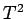tests to decide if any of the pairs are indistinguishable on the basis of the data here.

2. Assess whether there are any variables on which all 4 groups have means so similar that the variable in question will not really help discriminate. A hypothesis test with a large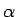is probably useful.

3. For the standard linear discriminant rule classify all of the data points and determine the raw and cross-validated error rates.

2. Fisher's iris data is available in S. Pick a pair of the 4 available variables and plot on a graph the boundaries of the linear and quadratic discriminant rules for discriminating the three varieties of iris along with the data. Estimate misclassification rates parametrically for both discriminant rules but, in estimating the error rates, do not assume that all the variance covariance matrices are the same.

3. From the text 11.28.

4. Consider the situation where you have two normal populations and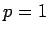; the populations are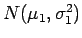and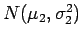. You have samples of size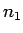and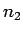and observe means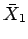and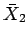where we assume that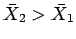. We plan to use the following rule:
R: Classify a new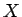as coming from population 2 if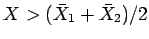We want to estimate the error rates for this classifier. The usual linear rule for known parameters would classifyin group 2 if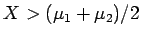and have an error rate of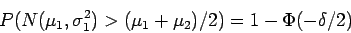where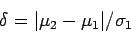NOTE: if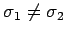then this linear rule is not the best rule but we are analyzing its performance when it is used in less than ideal circumstances.

1. Derive an expression for the conditional error rates givenandof rule R as a function of,,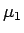and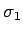.

2. Find a Taylor expansion for these quantities as a function ofandabout the pointsand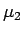. Keep terms out to quadratic in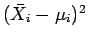.

3. Use this expression to compute the expected error rate and show that it is the error rate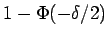for the linear classifier given above plus a term of the form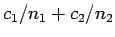. Your answer should include a formula for the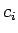.

4. The cross validation estimate of this error rate is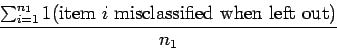Use the result in the first 3 parts of this question but with1 smaller get a formula for the expected value of this estimate plus terms like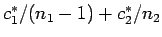.

5. Put these results together to argue that the expected value of the cross validation estimate of the error rates is the same, to terms of order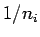as the expected error rate.

5. From the text questions 8.18 and 8.19. The data are in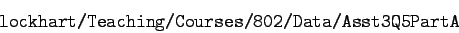6. From the text questions 9.28 and 9.29.

7. From the text 10.10.

8. From the text 12.16. CANCELLED.

Richard Lockhart
2002-11-20